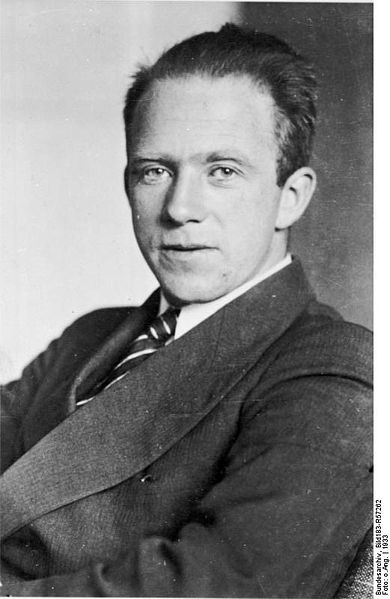# Heisenberg Uncertainty Principle

##$\LARGE{\Delta}x{\Delta}p\ge\hbar$

### The Terms${\Delta}x$ - The uncertainty in the position (in the x-direction) of an object [Units: m]${\Delta}p$ - The uncertainty in the momentum (in the x-direction) of an object [Units: kg m s-1]$\hbar$ - Planck's constant divided by$2\pi$  [Value: 1.051 × 10-34 m2 kg s-1]

### What Does It Mean?

This law states that particular pairs of physical properties, such as position and momentum, cannot be simultaneously known to arbitrarily high precision. This means that one cannot know the position of a particle exactly and also know its momentum or velocity exactly. Since the relation between energy and time in special relativity is the same as that between momentum and space. This relation is of central importance in quantum mechanics, and is a result of the quantum mechanical treatment of particles as wavepackets.

### Did you know?

The expression is often regarded as an experimental result concerning measurements of incompatible observables. In fact it can be derived theoretically, and is primarily a limit on the states that can be prepared. We cannot prepare a state with well defined position and momentum. Arguably,the famous EPR type experiments give a way to measure position and momentum simultaneously.

### Further information at Warwick

The Heisenberg Uncertainty Principle is introduced and used in the first year module "PX101 Quantum Phenomena" and features in many later modules, particularly in the areas of quantum mechanics and particle physics.